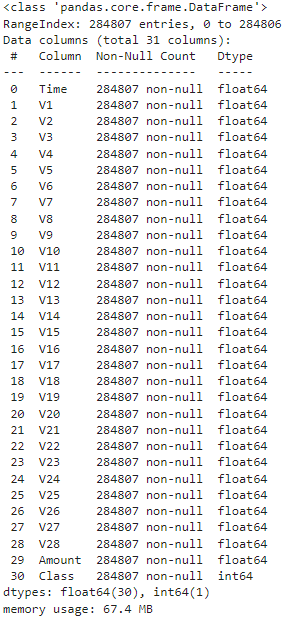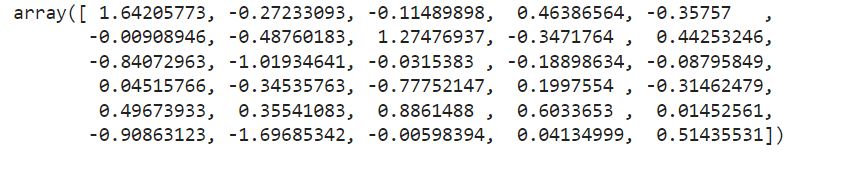top of page
• Hackers Realm

# Credit Card Fraud Detection using Python | Classification | Machine Learning Project Tutorial

Updated: Apr 15

Credit Card Fraud detection is one of the fundamental project of Machine Learning. This classification problem is similar to Titanic Data-Set Analysis and Iris Data-Set Analysis with different attributes. The objective is to detect Credit Card Fraud using Python.To calculate the model performance and to deal with this specific classification problem, we have to use different scoring metrics. Additionally, we will learn a few other techniques with the help of dataset.

You can watch the video-based tutorial with step by step explanation down below.

## Dataset Information

The dataset contains transactions made by credit cards in September 2013 by European cardholders. This dataset presents transactions that occurred in two days, where we have 492 frauds out of 284,807 transactions. The dataset is highly unbalanced, the positive class (frauds) account for 0.172% of all the transactions.

It contains only numerical input variables which are the result of a PCA transformation. Unfortunately, due to confidentiality issues, we cannot provide the original features and more background information about the data. Features V1, V2, … V28 are the principal components obtained with PCA, the only features which have not been transformed with PCA are 'Time' and 'Amount'. Feature 'Time' contains the seconds elapsed between each transaction and the first transaction in the dataset.

The feature 'Amount' is the transaction Amount, this feature can be used for example-dependant cost-sensitive learning. Feature 'Class' is the response variable and it takes value 1 in case of fraud and 0 otherwise.

Given the class imbalance ratio, we recommend measuring the accuracy using the Area Under the Precision-Recall Curve (AUPRC). Confusion matrix accuracy is not meaningful for unbalanced classification.

## Import modules

First, we have to import all the basic modules we will be needing for this project.

```import pandas as pd
import numpy as np
import seaborn as sns
import matplotlib.pyplot as plt
import warnings
warnings.filterwarnings('ignore')
%matplotlib inline```
• pandas - used to perform data manipulation and analysis.

• numpy - used to perform a wide variety of mathematical operations on arrays.

• matplotlib - used for data visualization.

• seaborn - built on top of matplotlib with similar functionalities.

• warnings - to manipulate warnings details filterwarnings('ignore') is to ignore the warnings thrown by the modules (gives clean results).

• %matplotlib - to enable the inline plotting.

```# load the csv data• Time attributes are in the terms of timestamp.

• The class attribute shows 0 indicates non fraudulent transactions and 1 indicates fraudulent transactions.

• The amount is in EUROs.

To display Statistical Information:

```# statistical info
df.describe()```• Due to the sizeable difference in the range of column (min) & (max), we need to run a standard scalar transformation later.

To Display DataType Information:

```# datatype info
df.info()```• All the input attributes(0-29) are floats and the output attribute(30) are in Integer.

## Preprocessing the dataset

Let us check for NULL values in the dataset.

```# check for null values
df.isnull().sum()```• There are no NULL values present in the dataset.

• If any NULL values are present, we have to fill all the NULL values before proceeding to model training.

## Exploratory Data Analysis

Let us first explore the categorical column "Class".

`sns.countplot(df['Class'])`• The number of fraudulent classes is low.

• Hence, we need to balance the data for reasonable results.

To display all the 28 PCA columns, we need to run a loop.

```df_temp = df.drop(columns=['Time', 'Amount', 'Class'], axis=1)

# create dist plots
fig, ax = plt.subplots(ncols=4, nrows=7, figsize=(20, 50))
index = 0
ax = ax.flatten()

for col in df_temp.columns:
sns.distplot(df_temp[col], ax=ax[index])
index += 1• Most columns show a bell curve (Normal Distribution), which does not require processing.

• If we have to deal with the basic algorithmic models (like Logistic Regression), we can also pass these data into Standard Scalar.

Let us explore the column "Time".

`sns.distplot(df['Time'])`To display the column "Amount".

`sns.distplot(df['Amount'])`• Due to the irregularities in range, we need to pass these data into Standard Scalar. Before that, we can have a look at Correlation Matrix.

## Correlation Matrix Analysis

In this specific project, the correlation matrix is insignificant because of the lack of meaningful information. All the columns containing random pieces of information is dynamically reduced using PCA transformation.

```corr = df.corr()
plt.figure(figsize=(30,40))
sns.heatmap(corr, annot=True, cmap='coolwarm')```• We can observe a correlation with respect to Amount.

• Usually, we had to drop a few columns in the correlation matrix. However, in this specific project, we can skip it.

## Input Split

```X = df.drop(columns=['Class'], axis=1)
y = df['Class']```
• Store the input attributes in variable X and output attribute in variable y

## Standard Scaling

```from sklearn.preprocessing import StandardScaler
sc = StandardScaler()
x_scaler = sc.fit_transform(X)```
`x_scaler[-1]`• All Input attributes are in the X and y contains the output Class.

• After running the code, we can see an array with a scaled value ranging from 0-1.

• To understand the process, please go through the formula of Standard Scalar.

## Model Training and Testing

To perform Model Training we will use several different models like Logistic Regression, Random Forest, XGBoost, etc., Before that, we need to split the data.

Splitting the Data:

```# train test split
from sklearn.model_selection import train_test_split
from sklearn.metrics import classification_report, f1_score
x_train, x_test, y_train, y_test = train_test_split(x_scaler, y, test_size=0.25, random_state=42, stratify=y)```
• We have to use stratify to uniformly distribute class variables (Because the class is not balanced).

Logistic Regression:

```from sklearn.linear_model import LogisticRegression
model = LogisticRegression()
# training
model.fit(x_train, y_train)
# testing
y_pred = model.predict(x_test)
print(classification_report(y_test, y_pred))
print("F1 Score:",f1_score(y_test, y_pred))```• Here, we can observe accuracy as 100% (Because of the Standard Scaling). However, the majority of the accuracy is based on Non-Fraudulent samples.

• F1-Score is a combination of Precision and Recall.

• Since the F1 score is around 72%, we have to consider a better Model for training.

Random Forest:

```from sklearn.ensemble import RandomForestClassifier
model = RandomForestClassifier()
# training
model.fit(x_train, y_train)
# testing
y_pred = model.predict(x_test)
print(classification_report(y_test, y_pred))
print("F1 Score:",f1_score(y_test, y_pred))```• After running the code, we have to wait longer than usual due to the larger number of Data-Set values.

• Now the F1-Score has improved.

• Due to unbalanced training, we are observing a low score.

• Let us try one boosting model.

XGBoost:

```from xgboost import XGBClassifier
model = XGBClassifier(n_jobs=-1)
# training
model.fit(x_train, y_train)
# testing
y_pred = model.predict(x_test)
print(classification_report(y_test, y_pred))
print("F1 Score:",f1_score(y_test, y_pred))```• We can observe an F1-Scor